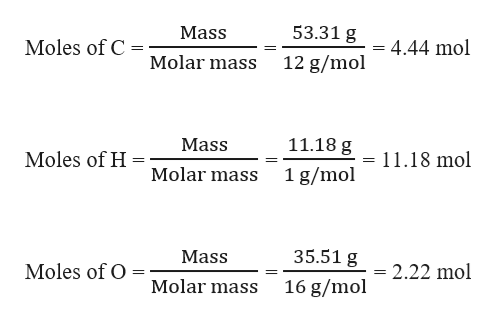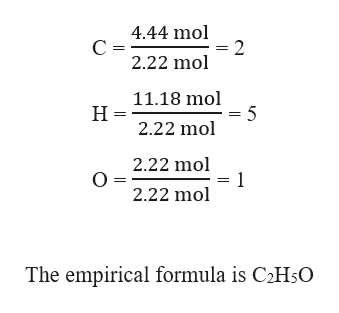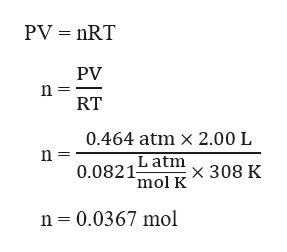# A 2.00 L tank contains an unknown gas that is 53.31% carbon, 11.18% hydrogen, and 35.51% oxygen by mass. At 35.0 °C and 0.470 bar, the gas in the tank has a mass of 3.30 g.What is the empirical formula of the gas?empirical formula:__________________________How many moles of gas are in the tank?moles of gas: _____________________ molWhat is the molecular formula of the gas?molecular formula: _________________

Question
273 views

A 2.00 L tank contains an unknown gas that is 53.31% carbon, 11.18% hydrogen, and 35.51% oxygen by mass. At 35.0 °C and 0.470 bar, the gas in the tank has a mass of 3.30 g.

What is the empirical formula of the gas?

empirical formula:__________________________

How many moles of gas are in the tank?

moles of gas: _____________________ mol

What is the molecular formula of the gas?

molecular formula: _________________

check_circle

Step 1

Given,

An unknown gas that is 53.31% carbon, 11.18% hydrogen, and 35.51% oxygen by mass. this means that 100 g of the gas contains:

Mass of carbon = 53.31 g

Mass of hydrogen = 11.18 g

Mass of oxygen = 35.51 g

Moles of C, H and O can be calculated as:help_outlineImage Transcriptionclose53.31 g Mass Moles of C 4.44 mol Molar mass 12 g/mol 11.18 g Mass Moles of H 11.18 mol Molar mass 1 g/mol 35.51 g Mass Moles of O = 2.22 mol Molar mass 16 g/mol fullscreen
Step 2

The empirical formula of the gas can be written by finding the simplest whole molar ratio as:help_outlineImage Transcriptionclose4.44 mol =2 2.22 mol С - 11.18 mol = 5 2.22 mol Н- 2.22 mol O = 2.22 mol 1 The empirical formula is C2H5O fullscreen
Step 3

Given,

Pressure, P = 0.470 bar = 0.464 atm      ( 1 bar = 1/1.013 atm)

Volume, V = 2.00 L

Temperature, T = 35.0 °C = (...help_outlineImage TranscriptionclosePV nRT PV n RT 0.464 atm x 2.00 L L atm 0.0821 x 308 K mol K n 0.0367 mol fullscreen

### Want to see the full answer?

See Solution

#### Want to see this answer and more?

Solutions are written by subject experts who are available 24/7. Questions are typically answered within 1 hour.*

See Solution
*Response times may vary by subject and question.
Tagged in

### General Chemistry